# A Deep Learning Model for Striae Identification in End Images of Float Glass

A Deep Learning Model for Striae Identification in End Images of Float Glass
State Key Laboratory of Metastable Materials Science and Technology, Yanshan University, Qinhuangdao 066004, China

North China Institute of Aerospace Engineering, Langfang 065000, China

Hebei CSG Glass Co., Ltd., 28 Baihe Road Yongqing Industrial Park, Langfang 065600, China

Corresponding Author Email:
lsm@ysu.edu.cn
Page:
85-93
|
DOI:
https://doi.org/10.18280/ts.370111
24 September 2019
|
Accepted:
3 January 2020
|
Published:
29 February 2020
| Citation

OPEN ACCESS

Abstract:

For float glass, there is a correlation between the striae in end image and the manufacturing process. If clearly understood, the correlation helps to optimize and fine-tune the manufacturing process of float glass. This paper attempts to extract the striae from the end image of float glass with deep learning (DL) neural network (NN). For this purpose, an image segmentation model was established based on improved U-Net, a fully convolutional network (FCN), and used to accurately divide the glass liquid on the end image into different layers. Firstly, the improved U-Net model was constructed to extract the striae from each liquid layer on the end image. Next, the activation function and convolutional mode of the improved U-Net model were optimized to enhance the segmentation accuracy and shorten the training/prediction time. Finally, the proposed model was tested on the float glass production line of Hebei CSG Glass Co., Ltd. The test results show that our model achieved an accuracy of 94%. The research findings lay a solid basis for striae identification on end image of float glass, and provide guidance for optimization and fine-tuning of float glass manufacturing process.

Keywords:

striae identification, end image, float glass, deep learning (DL), liquid layers, U-Net

1. Introduction

For float glass, there is a correlation between the striae in end image and the manufacturing process [1, 2]. If clearly understood, the correlation could greatly facilitate the diagnosis of manufacturing problems of float glass. For example, Liu  identified the basic structure of the end image of float glass (the glass liquid flowing out of the furnace has three layers, which respectively correspond to the three circulations in the furnace), and then proposed a regulation method for the melting process of float glass based on striae detection. Therefore, the homogenization failure of the glass liquid can be traced back to specific parts in the furnace, according to the striae on the end of float glass. In this way, the technological process of the furnace can be evaluated early and fine-tuned accurately to restore the stability and normality of float glass manufacturing, promoting the regulation of the melting process of float glass .

In general, the striae in end images of float glass are analyzed by experienced process experts. However, the expert analysis consumes lots of labor and time. Sometimes, the striae are difficult to identify or incorrectly identified, because of the experience difference between the experts and the limitations of their knowledge. The defects of expert analysis can be overcome by computer segmentation, which features high objectivity, fast data processing and good reproducibility. The various methods for image segmentation can be roughly divided into conventional methods and deep learning (DL) methods.

Each image contains three levels of semantics: low-level, intermediate-level and high-level. The low-level semantics (e.g. color, texture and shape) are adopted by the conventional image segmentation methods. Thresholding, region growing and edge detection [4, 5] are the most popular conventional methods for image segmentation. Yao  proposed an online identification method for glass surface defects, based on Otsu’s method and the Hessian blob algorithm. Li et al.  compensated the image under non-uniform illumination through top-hat transform of grayscale morphology, obtained a binary image by global image thresholding using Otsu’s method, and designed an algorithm for interconnected areas to optimize the features of low-contrast surface defects. However, the conventional image segmentation methods cannot achieve a good effect on the striae in the end image of float glass, owing to the discontinuous edges between liquid layers, the irregular shape of the image and the changing brightness with shooting devices.

Thanks to the development of DL theories, the DL-based techniques for image recognition, detection and semantic segmentation have gradually replaced the artificial strategies. Image semantic segmentation is an important research direction in computer vision and an essential part of image understanding. Convolutional neural network (CNN), a typical DL technique, provides a powerful tool for feature extraction. This technique has been successfully applied in various fields, namely, automatic pilot, medical image diagnosis, product defect detection, speech recognition, and agricultural product classification [8, 9]. Xiong et al.  developed a detection method for glass surface defects based on multiscale CNN, which can accurately identify the surface defects of glass, especially starches and impurities. Luo et al.  combined the improved dynamic thresholding and backpropagation neural network (BPNN) to detect the glass surface defects. Nevertheless, there is little report on DL-based striae detection in end image of float glass.

For the following reasons, it is very difficult to automatically segment the liquid flow in end image of float glass: (1) The end images captured by different striaescopes vary greatly from each other, under the effects of light intensity and irradiation uniformity; (2) In the end image of float glass, the liquid has obscure layers and disconnected edges, adding to the difficulty in striae detection; (3) There is no clear boundary between each liquid layer and the surrounding textures.

The accuracy of melting fault diagnosis is critical to the manufacturing efficiency of float glass, and the economic profit of the manufacturer. To achieve an accurate diagnosis, it is imperative to classify the glass liquid at the end into different layers automatically by computer technology. Drawing on the previous results on float glass melting, this paper introduces computer vision technique to classify the liquid layers and identify the striae in the end image. Based on the correspondence between liquid layers and circulations in the furnace, a series of end images with striae tags were combined into a dataset. On this basis, a neural network (NN) model was established and trained, and used to identify and diagnose the faults on the subsequent images.

Our research was carried out in the following steps: Firstly, the experts tagged the series of end images; the tagged information includes the layers of glass liquid and the manufacturing processes corresponding to the striae. Then, the original end images and the tagged data were integrated into a dataset. After that, a DL network model was set up, and the DL network was trained by the dataset to find the striae in the end images; the model structure and parameters were determined after repeated trainings and fine-tunings. Finally, the model with the selected structure and parameters was applied to predict and identify the striae in other end images of float glass.

The remainder of this paper is organized as follows: Section 2 introduces the data collection, data preprocessing and construction of the dataset; Section 3 develops a liquid layer segmentation method for end image of float glass based on improved U-Net, a fully convolutional network (FCN); Section 4 verifies the proposed method through several tests; Section 5 puts forward the conclusions.

2. Data Collection and Preprocessing

Our dataset covers 1,000 typical end images of float glass, which were collected from the float glass production line of Hebei CSG Glass Co., Ltd. To ensure the robustness and reliability of our model, the images were selected on different days under different manufacturing states. In each image, the striae on each liquid layer were tagged by experts. Then, the tagged data were put into our dataset, and used for model training. The data were collected and preprocessed in the following steps:

(1) Image collection

The end images were collected from float glass, using a self-developed striaescope. The triaescope consists of a light source system, a motor control system, an imaging system, and a computer software system. Specifically, the light source system generates parallel lights to irradiate the end surface of float glass sample; the motor control system performs logical control of the trolley carrying the sample; the imaging system shoots the end image of the sample with a charge-coupled device (CCD) lens and an optical lens; the computer software system coordinates the work of each system. Striaescopes have been adopted by hundreds of manufacturers around the world, because they can capture high-quality end images of glasses.

(2) Image normalization

The collected images often vary greatly in scale due to the difference in manufacturing device and glass thickness. However, the input data of the CNN must have the same dimension. Thus, the different images need to be converted into the same scale.

The float glass produced by Hebei CSG Glass Co., Ltd. is 4m-wide and 15mm-thick. The images collected by our striaescope were generally 300×4,000 in size. However, the image size might deviate from the standard size, under the effect of lens magnification of the imaging system. To solve the problem, all the images were normalized to 300×4,000. The normalization could distort the images and destroy their original features, because the images have different aspect ratios. Therefore, the images were resized with the short side as the benchmark, and the empty parts were filled with white color.

(3) Image tagging

The three layers of glass liquid on the normalized images were manually identified and tagged by experts. Since the glass liquid has three layers, the pixels on the middle layer were colored white, while those on the upper and lower layers were colored black. The tagged images were saved in the Masks folder with the same names of the original images, while the original images were all stored in the Images folder.

## 1.jpgFigure 1. Original end image

## 2.jpg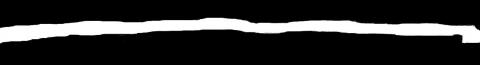Figure 2. Tagged end image

One of the original end images is presented in Figure 1, and the corresponding tagged image is displayed in Figure 2. As shown in Figure 2, the tagged end image is a binary image divided into three layers based on the features of glass liquid: the upper black layer, the middle white layer and the lower black layer. Thus, the division of liquid layers is a binary classification problem.

(4) Dataset division

The dataset was divided into a training set, a verification set and a test set at the ratio of 6:2:2. The training set (6,00 images) was used to train our model; the test set was used to verify the effect of the model trained by each batch of training images (the model structure was then adjusted based on the verification results); the test set was used to verify the effectiveness of the trained model.

(5) Data enhancement

The number of images in the training set is too few to prevent overfitting. This calls for data enhancement of the training set. To expand the data samples, the images in the training set were subjected to enhancement operations like translation, brightening, shading, scaling and adding salt-and-pepper noise. Neither rotation nor chrominance adjustment was adopted, because the layers of glass liquid depend on vertical positions and the original images are grayscale images. To ensure the randomness of enhanced data, the brightness level, shading position and scaling were generated randomly; the tags on the images to be translated and scaled were also subjected to translation and scaling; the tags on the images to be brightened or shaded were not changed.

First, 200 images were randomly selected from the 600 images in the training set. Each image was modified with 20 random brightening parameters. The brightening adds 4,000 images to the training set.

Second, 200 images were randomly selected from the training set. Each image was shaded in 10 random areas (the size and location of each area were randomly generated). The shading adds 2,000 images to the training set.

Third, 100 images were randomly selected from the training set. Each image was added with salt-and-pepper noises 9 times. The noise addition adds 900 images to the training set.

In this way, the size of the training set was expanded from 600 images to 7,500 images. The data in verification set and test set were not enhanced, for the two sets were used to evaluate the actual performance of our model. After data preprocessing, the authors obtained a training set of 7,500 images, a verification set of 200 images, and a test set of 200 images.

3. U-Net-Based Classification of Glass Liquid Layers

3.1 Structure of U-Net model

Each layer of glass liquid takes a specific texture, direction and form on the end image of float glass. There are clear boundaries between different layers, but the boundaries are sometimes discontinuous. Therefore, the different layers of glass liquid can be extracted through semantic segmentation. The popular ways of semantic segmentation include the FCN, SegNet, DeepLab [12, 13].

This paper adopts the U-Net, a typical FCN, for image segmentation. The FCN can realize pixel-level segmentation of images, providing a suitable tool for semantic segmentation. In classic CNN, a fixed-length eigenvector is outputted by the fully-connected layer after the convolutional layer, and then classified by SoftMax [14-16]. By contrast, the FCN can process image inputs of multiple dimensions. In the FCN, the feature maps from the convolutional layer are up-sampled in the deconvolution layer, and restored to the size of the original images. In this way, the spatial information of the original images is preserved, and each pixel can be predicted. Finally, the up-sampled feature maps are subjected to pixel-level classification.

The FCN-based image segmentation can be divided into two simple phases. In the first phase, each input image passes through a series of convolutional layers and pooling layers, which is similar to that of the CNN [17, 18]. The two kinds of layers reduce the spatial dimension of the image, and generate an abstract feature map in the light of local patterns. This process is also known as encoding.

In the second phase, the feature map from the encoder is up-sampled by a series of transposed convolutional layers (deconvolution layers), such that the feature map has the same size as the input image. This process is also known as decoding. Figure 3 shows the height H and width W of the feature map after passing through each layer in the FCN.

## 3.png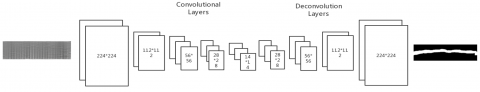Figure 3. Structure of the FCN

The decoding phase outputs an H ×W×C feature map, where C is the hyper-parameter. Then, the C channel is combined with n channels on the level of pixels, where n is the number of object categories. The pixel-level feature fusion is realized through dimensionality reduction by a 1×1 kernel.

The FCN is the first DL-based image segmentation method. Its merit lies in the realization of end-to-end segmentation [19, 20]. However, the details of FCN image segmentation are not good enough, for failing to consider the spatial correlation between pixels. To refine the details, this paper improves the U-Net (Figure 4).

## 4.png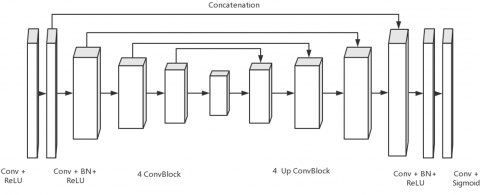Figure 4. Structure of the U-Net

As shown in Figure 4, the U-Net consists of two parts: the feature extraction part on the left, and the up-sampling part on the right. The former part contains a series of convolutional layers and pooling layers. The feature map changes to a new dimension after passing through each pooling layer. In total, there are 5 dimensions in the former part. The latter part includes a series of deconvolution layers. After each up-sampling, the dimension of the feature map is fused (stitched) with that of the feature map in the corresponding channel of the former part.

3.2 Improved U-Net

In the CNN, the area on the original image that corresponds to the pixels on the output feature map is called the receptive field. The larger the kernel is, the wider the receptive field, and the richer the output information. Therefore, large kernels are suitable for extracting features from large regions [21-23].

In the end image of float glass, each stria runs through the entire image from left to right, creating a large feature region. Large kernels could work effectively on such a large feature region. Nevertheless, large kernels increase the number of training parameters, which consume a high computing power and hinders model convergence. To overcome the defects, this paper introduces dilated convolution to the U-Net.

Dilated convolution is produced by adding holes with zero weight to the standard convolution. These holes do not participate in the convolutional operation [24, 25]. The addition of these holes can expand the receptive field of the kernel without increasing the number of parameters.

Three kinds of 3×3 kernels are presented in Figure 5. In Figure 5(a), the kernel has an expansion ratio of 1, and a receptive field of 3×3; this kernel is equivalent to a standard kernel. Figure 5(b) shows a perforated kernel with an expansion ratio of 2 and a receptive field of 5×5; only the black elements are involved in convolutional operation, i.e. all elements are of zero weight except the nine black ones. Figure 5(c) presents a perforated kernel with an expansion ratio of 3 and a receptive field of 7×7.

The receptive field of a kernel increases with expansion ratio. However, a larger expansion ratio is not necessarily better. Dilated convolution performs sparse convolutional operation on images. If the expansion ratio is too large, the kernel will emphasize on global information over local details.

## 5a.png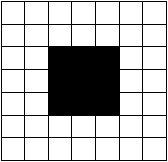(a) Expansion ratio=1

## 5b.jpg(b) Expansion ratio=2

## 5c.jpg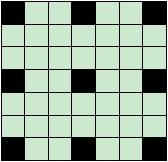(c) Expansion ratio=3

Figure 5. Perforated kernels

The proposed dilated U-Net provides a contraction path to extract image features and an expansion path to restore to the size of original image. Unlike the original U-Net, the dilated U-Net replaces the standard kernels with perforated ones. To prevent the vanishing gradient problem, batch normalization (BN) layers were added to normalize the convolutional outputs in batches, thus enhancing gradient and speeding up convergence.

## 6.png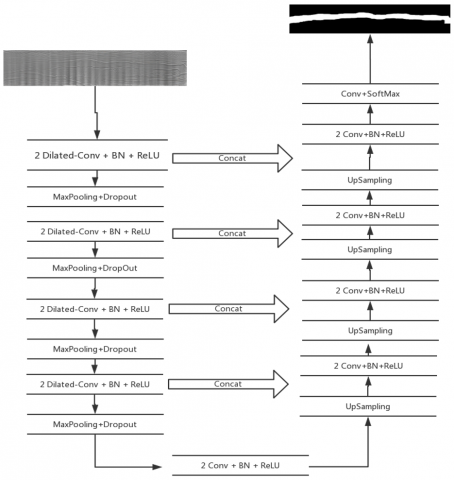Figure 6. Structure of dilated U-Net

Note: Dilated-CONV is dilated convolution; BN is batch normalization; ReLU is the nonlinear activation function; MaxPooling is the pooling layer; UpSample is up-sampling; Concate is information fusion (concatenation), i.e. the corresponding feature maps on the contraction and expansion paths are superimposed to integrate contextual features; SoftMax is the classifier that normalizes multiple neuron outputs to (0, 1), i.e. the probabilities the input image belongs to different categories.

As shown in Figure 6, the multi-scale structure is suitable for segmenting ultra-large images. Hence, the dilated U-Net applies well to the classification of glass liquid layers. However, two more problems must be solved: the high computing load and over-fitting. The former problem arises from the numerous training parameters, which are resulted from the large scale of end images; the latter problem is attributable to the small training set.

To solve the problems, a dropout layer was added to each pooling layer in the dilated U-Net. The basic idea of dropout is as follows: During the training, some neurons are filtered out from each batch at a random probability; only the parameters corresponding to the remaining neurons are trained . The removed neurons have a gradient of zero, and their parameters will not be updated. However, all the neurons should participate in the computation during the test phase. Here, a total of 485,673 parameters need to be trained, at the dropout ratio of 0.5.

During the training, the initial learning rate was set to 1×10-5, the weight attenuation rate to 1×10-4, and the momentum parameter to 0.85. The kernels were initialized with Gaussian distribution N(0, 0.1). The improved U-Net was optimized by stochastic gradient descent (SGD). The “stochastic” means a part of dataset is stochastically selected for computation, i.e. a batch version of gradient descent. The SGD supports the momentum parameter and learns the attenuation rate.

Instead of computing the loss on all training data, the SGD algorithm calculates the loss based on a randomly selected part of the data [27, 28]. This speeds up the parameter update in each iteration. However, a small loss in some data does not necessarily mean the loss in all data is small. As the result, the SGD algorithm sometimes cannot converge to the global optimal solution . Considering the memory limit of computer and experimental results, the authors decided to contain 32 images in each batch of training samples, aiming to clarify the direction of gradient descent, control training oscillations and reduce the number of iterations.

The image segmentation problem is actually a binary classification of each pixel. Thus, the logarithmic loss function, i.e. binary cross entropy function, was adopted as the objective function . For the binary classification problem, there are two possible categories for each image. Let p and 1-p be the probabilities for the image to fall into the two categories, respectively. Then, the objective function can be defined as:

$\text{L}=-\left[ y*\log (p)+(1-y)*\log (1-p) \right]$

where, y is the label of each sample (y=1 for the positive category; y=0 for the negative category); p is the probability that a sample is predicted as a positive one.

3.3 Evaluation indices

The image segmentation effect of the improved U-Net was evaluated by the metrics for binary classification, namely, accuracy, error rate, sensitivity, specificity, recall, precision and F-measure. Let true positive (TP) be the number of positive samples predicted as positive; true negative (TN) be the number of negative samples predicted as negative; false positive (FP) be the number of negative samples predicted as positive; false negative (FN) be the number of positive samples predicted as negative. Then, the six evaluation indices can be described as follows:

(1) Accuracy

This common evaluation index describes the proportion of correctly classified samples. The higher the accuracy, the better is the effect of image segmentation. The accuracy can be calculated by:

$\text{Accuracy}=\frac{TP+TN}{TP+TN+FP+FN}$

(2) Error rate

Contrary to accuracy, the error rate refers to the proportion of incorrectly classified samples:

$\text{Error}Rate=\frac{FP+FN}{TP+TN+FP+FN}$

The error rate and the accuracy are mutually exclusive events. Hence, it is only necessary to compute the accuracy.

(3) Sensitivity

This metric represents the proportion of correctly classified positive samples, and measures the recognition ability of the classifier of positive samples. The sensitivity can be calculated by:

$\text{Sensitivity}=\frac{TP}{P}$

(4) Specificity

This metric represents the proportion of correctly classified negative samples, and measures the recognition ability of the classifier of negative samples. The specificity can be calculated by:

$\text{Specificity}=\frac{TN}{N}$

(5) Recall

Being a measure of coverage, recall reflects how many positive samples are classified as positive. This index is the same as sensitivity.

(6) Precision

Precision refers to the proportion of correctly classified positive samples:

$\text{Precision}=\frac{TP}{TP+FP}$

(7) F-measure

The F-measure is the weighted harmonic mean of accuracy and recall:

$\text{F}=\frac{({{\text{ }\!\!\alpha\!\!\text{ }}^{\text{2}}}+1)P*R}{{{\text{ }\!\!\alpha\!\!\text{ }}^{\text{2}}}(P+R)}$

where, α=1. The F-measure is the combined result of accuracy and recall. The improved U-Net is effective if the F-measure is high.

4. Experimental Verification

4.1 Experimental environment

Our DL model operates under the DL frameworks of TensorFlow and Keras. The CNN was trained on Nvidia Tesla v100 GPU, and data enhancement module was processed on OpenCV, a library of programming functions, under Python 3.6. The areas of the three liquid layers were computed by the find Contour in OpenCV.

4.2 Experimental results

A total of 1,000 end images of float glass were selected and divided into a training set, a verification set and a test set. The training set was subjected to data enhancement, and expanded to 7,500 images. These images were imported to the improved U-Net for training. The accuracies and losses of the training set and validation set after 35 rounds of training are shown in Figures 7 and 8, respectively.

## 7.jpg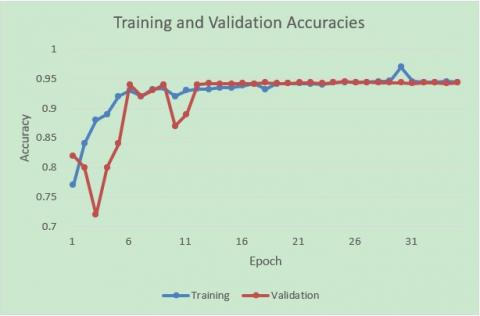Figure 7. The accuracy curves of training and validation sets

## 8.jpg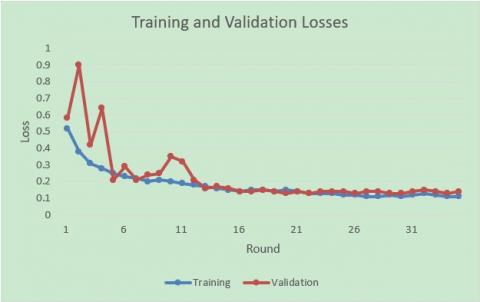Figure 8. The loss curves of training and validation sets

As shown in Figure 7, the accuracy of our model on the training set was on the rise after 35 rounds of training, but did not increase on the verification set after 20 rounds. The loss curves in Figure 8 indicate that the loss function continued to decline on the training set, but stopped decreasing on the verification set after 20 rounds. It can be concluded that, after 20 rounds of training, the network suffered from over-fitting, and the final accuracy of our model was about 94%. Therefore, the parameters after 20 rounds of training were taken as the final parameters of our model.

To verify the performance of our model in classifying gas liquid layers, the manual segmentation results of end images on float glass were taken as the reference, and the mean values of accuracy, F-measure and precision were computed based on the automatic segmentation results on 200 test images. The results of the improved U-Net were compared with those of the classic image segmentation methods, namely, edge detection, FCN and classic U-Net. As shown in Table 1, the improved U-Net outperformed the three contrastive models; On the 200 test images, our model achieved an accuracy of 94%, an F-measure was 79% and a precision of 81%.

The performance of our model in classifying gas liquid layers was further evaluated against the FCN and classic U-Net, based on the mean Dice coefficient (DC) and intersection over union (IoU) on automatic segmentation results on 200 test images. DC describes the ratio of the overlapping area between automatic segmentation and manual segmentation to the total area. The IoU is the intersection over union between the areas of automatic segmentation and manual segmentation. In ideal scenario, the intersection and union completely overlap each other, and the IoU equals 1. The DC and IoU values in Table 2 demonstrate that the improved U-Net achieved good segmentation accuracy, shedding new light on liquid layer classification of end images of float glass.

Table 1. Comparison of image segmentation results

 Accuracy F-measure Precision Edge detection 0.63202 0.61232 0.7233 FCN 0.84234 0.72132 0.8120 U-Net 0.91784 0.7556 0.7921 Improved U-Net 0.94151 0.79213 0.8143

Table 2. Comparison of different metrics

 Metrics FCN U-Net Improved U-Net DC 0.8323 0.9123 0.9432 IoU 0.8146 0.9103 0.9384

To validate the prediction effect, the improved U-Net was applied to segment two randomly selected end images of float glass (Figures 9a and 9c), which differ in striae thickness. The prediction results of the improved U-Net on the random images are displayed in Figures 9b and 9d.

## 9.jpg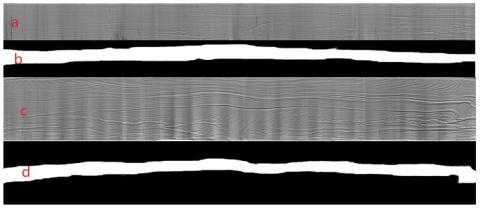Figure 9. Prediction results on random images

As shown in Figure 9, the improved U-Net classified the glass liquid into three layers. The area ratio between the three layers was 11:19:24 and 18:10:23 in the two end images. This ratio helps to reveal the technical features of float glass. In Figure 9a, the first layer takes up a small portion and obeys a non-uniform distribution. This means occlusions may exist in the glass liquid. In Figure 9c, the third layer accounts for a large portion, a sign of large tensile stress. Under large tensile stress, the glass products have a relatively low quality and grade, despite the high output. The manufacturer should adjust the tensile stress to strike a balance between output, quality and economic benefit. Moreover, the same defect in different layers corresponds to different technical parameters. The classification of glass liquid layers lays a solid basis for the diagnosis on the correspondence between end images and techniques of float glass.

5. Conclusions

This paper applies a DL model to classify the liquid layers on end images of float glass, and establishes an image recognition system that characterizes the homogenization quality of float glass.

Firstly, the U-Net model was adopted to extract the image features of liquid flow on different layers. This model was selected for several reasons. First, the multi-scale structure brings good feature expression ability. Next, the U-Net supports training with input images of different scales, and thus tolerates the inconsistent size of images on different types of glasses. To promote generalization ability, the small dataset was expanded by data enhancement. In addition, the dropout layers were added to the U-Net to reduce the number of training parameters, shorten the training and prediction time and improve the model availability.

The improved U-Net was applied to segment 100 actual end images of float glass in the test set. The accuracy, F-measure and precision reached 94%, 79% and 81%, respectively. The experimental results show that the improved U-Net satisfies the demand for actual production, and visually displays the technical features of float glass, laying a solid basis for the diagnosis of float glass production techniques.

Acknowledgment

This work was supported by The National Key Research and Development Program of China (2016YFB0303700).

References

 Feng, Z., Li, D., Qin, G., Liu, S. (2008). Study of the float glass melting process: Combining fluid dynamics simulation and glass homogeneity inspection. Journal of the American Ceramic Society, 91(10): 3229-3234. https://doi.org/10.1111/j.1551-2916.2008.02606.x

 Feng, Z., Li, D., Qin, G., Liu, S. (2009). Effect of the flow pattern in a float glass furnace on glass quality: calculations and experimental evaluation of on-site samples. Journal of the American Ceramic Society, 92(12): 3098-3100. https://doi.org/10.1111/j.1551-2916.2009.03319.x

 Liu, S.M. (2010). Technology of float glass melting process for stripe monitoring. Journal of Wuhan University of Technology, 32(22): 92-101. https://doi.org/10.3963/j.issn.1671-4431.2010.22.024

 Wei, S.S., Zhang, H., Wang, C., Wang, Y.Y., Xu, L. (2019). Multi-temporal SAR data large-scale crop mapping based on U-Net model. Remote Sensing, 11(1): 68. https://doi.org/10.3390/rs11010068

 Wang, C., Zhao, Z.Y., Ren, Q.Q., Xu, Y.T., Yu, Y. (2019). Dense U-net based on patch-based learning for retinal vessel segmentation. Entropy, 21(2): 168. https://doi.org/10.3390/e21020168

 Yao, Z.H. (2018). Discussion on online detection technology of glass production defects. Fujian Chemical Industry, 2018(5): 264-265, 271.

 Li, C.Y., Liu, Z., Li, S.T. (2018). Low-contrast surface defect detection algorithm for flat glass. Mechanical Engineer, 2018(3): 21-23. https://doi.org/10.3969/j.issn.1002-2333.2018.03.008

 Zhu, H., Shi, F., Wang, L., Hung, S.C., Chen, M.H., Wang, S., Lin, W.L., Shen, D. (2019). Dilated dense U-net for infant hippocampus subfield segmentation. Frontiers in Neuroinformatics, 13: 30. https://doi.org/10.3389/fninf.2019.00030

 Jung, H.Y., Lee, K.M. (2015). Image segmentation by edge partitioning over a nonsubmodular Markova random field. Mathematical Problems in Engineering, 2015: 1-9. https://doi.org/10.1155/2015/683176

 Xiong, H.L., Fan, C.Q., Zhao, S., Yu, Y. (2019). Detection method of glass surface defects based on multi-scale convolution neural network. Computer Integrated Manufacturing Systems, 2019: 1-16. http://kns.cnki.net/kcms/detail/11.5946.tp.20190708.1137.014.html.

 Luo, C., Gao, J., Sha, F.Y., Luo, F. (2016). Research on on-line defect detection system based on machine vision. Digital Technology and Application, 2016(4): 46-48.

 Kumar, K.S., Venkatalakshmi, K., Karthikeyan, K. (2019). Lung cancer detection using image segmentation by means of various evolutionary algorithms. Computational and Mathematical Methods in Medicine, 2019: 4909846. https://doi.org/10.1155/2019/4909846

 Liu, C.C., Zhang, Y.C., Chen, P.Y., Lai, C.C., Chen, Y.H., Cheng, J.H., Ko, M.H. (2019). Clouds classification from sentinel-2 imagery with deep residual learning and semantic image segmentation. Remote Sensing, 11(2): 119. https://doi.org/10.3390/rs11020119

 Hržić, F., Štajduhar, I., Tschauner, S., Sorantin, E., Lerga, J. (2019). Local-entropy based approach for X-Ray image segmentation and fracture detection. Entropy, 21(4): 338. https://doi.org/10.3390/e21040338

 Wu, M.H., Wang, Q., Rigall, E., Li, K.G., Zhu, W.B., He, B., Yan, T.H. (2019). ECNet: Efficient convolutional networks for side scan sonar image segmentation. Sensors, 19(9): 2009. https://doi.org/10.3390/s19092009

 Zheng, Q., Wu, Y., Fan, Y. (2018). Integrating semi-supervised and supervised learning methods for label fusion in multi-atlas based image segmentation. Frontiers in Neuroinformatics, 12: 69. https://doi.org/10.3389/fninf.2018.00069

 Ma, B., Ban, X., Huang, H., Chen, Y., Liu, W., Zhi, Y. (2018). Deep learning-based image segmentation for Al-La alloy microscopic images. Symmetry, 10(4): 107. https://doi.org/10.3390/sym10040107

 Ren, Y., Zhu, C., Xiao, S. (2018). Object detection based on fast/faster RCNN employing fully convolutional architectures. Mathematical Problems in Engineering, 2018: 1-7. https://doi.org/10.1155/2018/3598316

 Skovsen, S., Dyrmann, M., Mortensen, A., Steen, K., Green, O., Eriksen, J., Gislum, R., Jorgensen, R.N., Karstoft, H. (2017). Estimation of the botanical composition of clover-grass leys from RGB images using data simulation and fully convolutional neural networks. Sensors, 17(12): 2930. https://doi.org/10.3390/s17122930

 Al-Bander, B., Williams, B., Al-Nuaimy, W., Al-Taee, M., Pratt, H., Zheng, Y. (2018). Dense fully convolutional segmentation of the optic disc and cup in colour fundus for glaucoma diagnosis. Symmetry, 10(4): 87. https://doi.org/10.3390/sym10040087

 Wang, X.B., Li, A.J., Ci, Q.P., Shi, M., Jing, T.L., Zhao, W.Z. (2019). The study on tire tread depth measurement method based on machine vision. Advances in Mechanical Engineering, 11(4): 168781401983782. https://doi.org/10.1177/1687814019837828

 Zhou, Q.B., Chen, R.W., Huang, B., Liu, C., Yu, J., Yu, X.Q. (2019). An automatic surface defect inspection system for automobiles using machine vision methods. Sensors, 19(3). 644. https://doi.org/10.3390/s19030644

 Ozluoymak, O.B., Bolat, A., Bayat, A., Guzel, E. (2019). Design, development, and evaluation of a target oriented weed control system using machine vision. Turkish Journal of Agriculture and Forestry, 43(2): 164-173. https://doi.org/10.3906/tar-1803-8

 Lei, X., Ouyang, H., Xu, L. (2019). Mature pomegranate recognition methods in natural environments using machine vision. Ciência Rural, 49(9): https://doi.org/10.1590/0103-8478cr20190298

 Zhang, X., Zhang, J., Ma, M., Chen, Z., Yue, S., He, T., Xu, X. (2018). A high precision quality inspection system for steel bars based on machine vision. Sensors, 18(8): 2732. https://doi.org/10.3390/s18082732

 Hong, Y., Chang, B., Peng, G., Yuan, Z., Hou, X., Xue, B., Du, D. (2018). In-process monitoring of lack of fusion in ultra-thin sheets edge welding using machine vision. Sensors, 18(8): 2411. https://doi.org/10.3390/s18082411

 El-Faki, M.S., Song, Y.Q., Zhang, N.Q., El-Shafie, H.A., Xin, P. (2018). Automated detection of parasitized Cadra cautella eggs by Trichogramma bourarachae using machine vision. International Journal of Agricultural and Biological Engineering, 11(3): 94-101. https://doi.org/10.25165/j.ijabe.20181103.2895

 Wang, Y.X., Xu, S.S., Li, W.B., Kang, F., Zheng, Y.J. (2017). Identification and location of grapevine sucker based on information fusion of 2D laser scanner and machine vision. International Journal of Agricultural and Biological Engineering, 10(2): 84-93. https://doi.org/10.3965/j.ijabe.20171002.2489

 Türkcan, S., Naczynski, D.J., Nolley, R., Sasportas, L.S., Peehl, D.M., Pratx, G. (2016). Endoscopic detection of cancer with lensless radioluminescence imaging and machine vision. Scientific Reports, 6(1): 30737. https://doi.org/10.1038/srep30737

 Sanchez-Romero, A., González, J.A., Calbó, J., Sanchez-Lorenzo, A. (2015). Using digital image processing to characterize the Campbell–Stokes sunshine recorder and to derive high-temporal resolution direct solar irradiance. Atmospheric Measurement Techniques, 8(1): 183-194. https://doi.org/10.5194/amt-8-183-2015